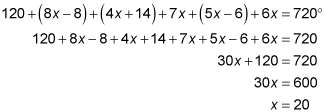# INTERIOR ANGLES SUM ASSIGNMENT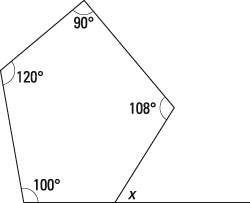Solve for x.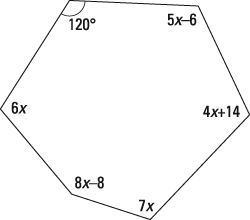1. 58 degrees

The sum of the exterior angles of a polygon is 180 (n – 2), where n represents the number of sides. The sum of the angles of a pentagon (five sides) is equal to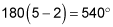The pentagon is missing one interior angle, which you can call y: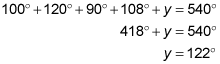The interior and exterior angles of a polygon are supplementary. Therefore,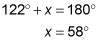2. 20 degrees

The sum of the interior angles of a polygon is 180 (n – 2), where n represents the number of sides. The sum of the angles of a hexagon (six sides) is equal to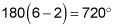Add the interior angles, set the sum equal to 720, and solve for x: